Next: An alternative: the single Up: Mathematical background Previous: Principle   Contents

Managing the bisection and ordering

The second problem is solved in the following way: assume that at some step of the algorithm the bisection process leads to the creation of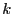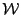such that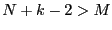. As we have previously considered the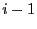elements of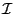we may use them as storage space. This means that we will store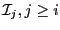at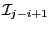thereby freeingelements. In that case the procedure will fail only if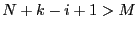.

Now we have to manage the ordering of the. We have defined two types of order for a given set of boxes:

1. maximum equation ordering: the box are ordered along the value of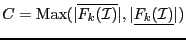for allin [1,]. The first box will have the lowest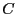.
2. maximum middle-point equation ordering: let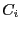be the vector whose components are the middle points of the intervals. The box are ordered along the value of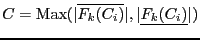for allin [1,]. The first box will have the lowest.
When adding thewe will substitute the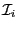by thehaving the lowestwhile the otherswill be added to the listby increasing order of. The purpose of these ordering is to try to consider first the box having the highest probability of containing a solution. This ordering may have an importance in the determination of the solution intervals (see for example section 2.3.5.2).

This method of managing the bisection is called the Direct Storage mode and is the default mode in ALIAS. But there is another mode, called the Reverse Storage mode. In this mode we still substitute theby thehaving the lowestbut instead of adding the remainingat the end of the listwe shift bythe boxes in the list, thereby freeing the storage of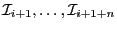which is used to store the remaining. In other words we may consider the solving procedure as finding a leaves in a tree which are solutions of the problem: in the Direct Storage mode we may jump during the bisection from one branch of the tree to another while in the Reverse storage mode we examine all the leaves issued from a branch of the tree before examining the other branches of the tree. If we are looking for all the solutions the storage mode has no influence on the total number of boxes that will be examined. But the Reverse Storage mode may have two advantages:

• if we are looking for only one solution it may enable to find it more rapidly (but that is not compulsory, see section 2.3.5.4),
• as we are following one branch at a time we will consider very rapidly small box that either will lead to a solution or will be discarded thereby enabling to free some storage space. Hence the storage space available in the reverse mode will be in general higher than in the direct mode: a practical consequence is that a problem may not be solved with the direct mode due to problem in the storage while with the same amount of storage solutions will be obtained in the reverse mode.
To switch the storage mode see section 2.3.4.5 .We may also define a mixed strategy which is staring in the direct mode and then switching to the reverse mode when the storage becomes a problem (see section 8.3).Next: An alternative: the single Up: Mathematical background Previous: Principle   Contents
Jean-Pierre Merlet 2012-12-20